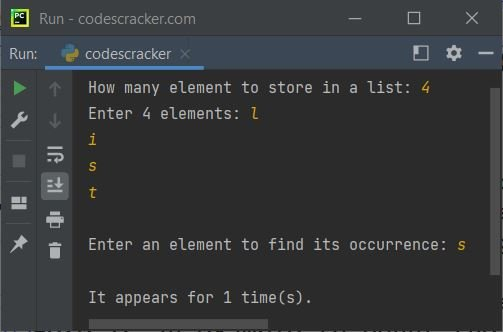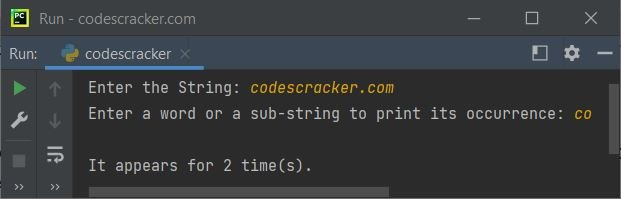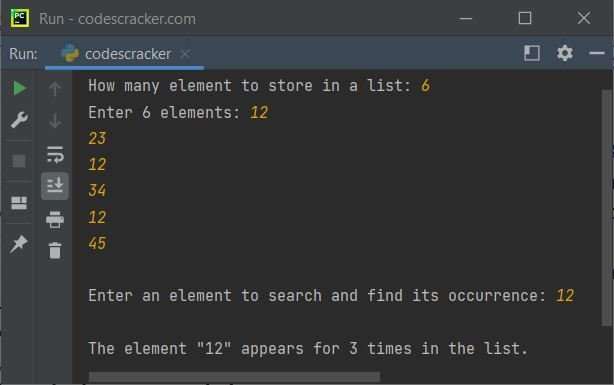# Python count() Function

The count() function in Python returns the number of occurrence of a particular element or specified sub-string in a list or a string. For example:

```mylist = [43, 54, 679, 32]
print(mylist.count(54))

mystring = "codes cracker dot com"
print(mystring.count("dot"))```

The output produced by above Python program, demonstrating the count() function, is:

```1
1```

because the element 54 appears for one time in the list named a, whereas the sub-string "dot" also appears for 1 time in the string named mystring. Therefore the output was 1 and 1 for both the use of count().

## Python count() Function Syntax

The syntax to use the count() function in Python is:

`var.count(value)`

As already told that the count() function returns the total number of times, a value (an element of a list or a sub-string of a string) available in a list or a string. Therefore, if the value passed as an argument of count() is not available in the list or string, then the function returns 0. Meaning that, the given value appears 0 times in the list or string.

## Python count() Function Example

Here is an example to count the occurrence of a value available in a list, entered by user:

```print("How many element to store in a list: ", end="")
n = int(input())
print("Enter", n, "elements: ", end="")
nums = []
for i in range(n):
nums.append(input())

print("\nEnter an element to find its occurrence: ", end="")
element = input()
print("\nIt appears for", nums.count(element), "time(s).")```

The snapshot given below shows the sample run of above Python program, with user input 4 as number of items to store in the list. l, i, s, t as four elements, and s as element to find and print its occurrence:Here is another example of count() function, to count the occurrence of a sub-string in a string, entered by user at run-time of the program:

```print("Enter the String: ", end="")
mystr = input()
print("Enter a word or a sub-string to print its occurrence: ", end="")
wrd = input()

tot = mystr.count(wrd)
print("\nIt appears for", tot, "time(s).")```

Here is its sample run with user input codescracker.com as string and co as sub-string to find and print its occurrence:That is, "codescracker.com" are the two "co" available in the string.

### Python count() Function - Main Use

The main use of count() function in Python, is to either search for an element or to search and find the number of occurrence of an element in a list. Here is an example:

```print("How many element to store in a list: ", end="")
n = int(input())
print("Enter", n, "elements: ", end="")
nums = []
for i in range(n):
nums.append(input())

print("\nEnter an element to search and find its occurrence: ", end="")
element = input()
x = nums.count(element)
if x == 0:
print("\nThe element \"", element, "\" is not available in the list.", int(input(")
elif x == 1:
print("\nThe element \"", element, "\" appears once in the list.", int(input(")
else:
print("\nThe element \"", element, "\" appears for ", x, " times in the list.", int(input(")```

Sample run of above program, with user input 6 as list size, and 12, 23, 12, 34, 12, 45 as six elements and 12 as element to search and counts its occurrence, is shown in the snapshot given below:The same things happens in case of a string. That is, the function named count() returns the occurrence of a particular word or a sub-string in a string.

Python Online Test

« Previous Function Next Function »